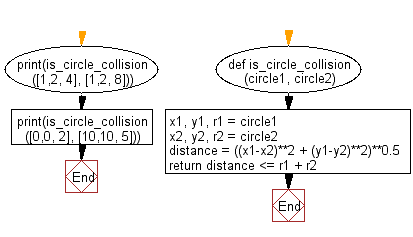﻿ Python: Check whether two given circles are intersecting - w3resource

# Python: Check whether two given circles are intersecting

## Python Basic - 1: Exercise-111 with Solution

Write a Python program which checks whether two circles in the same plane (with the same center (x,y) and radius) intersect. If intersection occurs, return true, otherwise return false.

Sample Solution:

Python Code:

``````def is_circle_collision(circle1, circle2):
x1, y1, r1 = circle1
x2, y2, r2 = circle2
distance = ((x1-x2)**2 + (y1-y2)**2)**0.5
return distance <= r1 + r2
print(is_circle_collision([1,2, 4], [1,2, 8]))
print(is_circle_collision([0,0, 2], [10,10, 5]))
``````

Sample Output:

```True
False
```

Flowchart:Python Code Editor:

Have another way to solve this solution? Contribute your code (and comments) through Disqus.

What is the difficulty level of this exercise?

Test your Programming skills with w3resource's quiz.

﻿